# The Universe of Discourse

Sun, 18 Jun 2006
 BuyPrincipia Mathematica (through section 56)from Bookshop.org(with kickback)(without kickback)
Whitehead and Russell's Principia Mathematica is famous for taking a thousand pages to prove that 1+1=2. Of course, it proves a lot of other stuff, too. If they had wanted to prove only that 1+1=2, it would probably have taken only half as much space.

Principia Mathematica is an odd book, worth looking into from a historical point of view as well as a mathematical one. It was written around 1910, and mathematical logic was still then in its infancy, fresh from the transformation worked on it by Peano and Frege. The notation is somewhat obscure, because mathematical notation has evolved substantially since then. And many of the simple techniques that we now take for granted are absent. Like a poorly-written computer program, a lot of Principia Mathematica's bulk is repeated code, separate sections that say essentially the same things, because the authors haven't yet learned the techniques that would allow the sections to be combined into one.

For example, section ∗22, "Calculus of Classes", begins by defining the subset relation (∗22.01), and the operations of set union and set intersection (∗22.02 and .03), the complement of a set (∗22.04), and the difference of two sets (∗22.05). It then proves the commutativity and associativity of set union and set intersection (∗22.51, .52, .57, and .7), various properties like !!\alpha\cap\alpha = \alpha!! (∗22.5) and the like, working up to theorems like ∗22.92: !!\alpha\subset\beta \rightarrow \alpha\cup(\beta - \alpha)!!.

Section ∗23 is "Calculus of Relations" and begins in almost exactly the same way, defining the subrelation relation (∗23.01), and the operations of relational union and intersection (∗23.02 and .03), the complement of a relation (∗23.04), and the difference of two relations (∗23.05). It later proves the commutativity and associativity of relational union and intersection (∗23.51, .52, .57, and .7), various properties like !!\alpha\dot\cap\alpha = \alpha!! (∗22.5) and the like, working up to theorems like ∗23.92: !!\alpha\dot\subset\beta \rightarrow \alpha\dot\cup(\beta \dot- \alpha)!!.

The section ∗24 is about the existence of sets, the null set !!\Lambda!!, the universal set !!{\rm V}!!, their properties, and so on, and then section ∗24 is duplicated in ∗25 in a series of theorems about the existence of relations, the null relation !!\dot\Lambda!!, the universal relation !!\dot {\rm V}!!, their properties, and so on.

That is how Whitehead and Russell did it in 1910. How would we do it today? A relation between S and T is defined as a subset of S × T and is therefore a set. Union, intersection, difference, and the other operations are precisely the same for relations as they are for sets, because relations are sets. All the theorems about unions and intersections of relations, like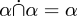, just go away, because we already proved them for sets and relations are sets. The null relation is the null set. The universal relation is the universal set.

A huge amount of other machinery goes away in 2006, because of the unification of relations and sets. Principia Mathematica needs a special notation and a special definition for the result of restricting a relation to those pairs whose first element is a member of a particular set S, or whose second element is a member of S, or both of whose elements are members of S; in 2006 we would just use the ordinary set intersection operation and talk about R ∩ (S×B) or whatever.

Whitehead and Russell couldn't do this in 1910 because a crucial piece of machinery was missing: the ordered pair. In 1910 nobody knew how to build an ordered pair out of just logic and sets. In 2006 (or even 1956), we would define the ordered pair <a, b> as the set {{a}, {a, b}}. Then we would show as a theorem that <a, b> = <c, d> if and only if a=c and b=d, using properties of sets. Then we would define A×B as the set of all p such that p = <a, b> ∧ aAbB. Then we would define a relation on the sets A and B as a subset of A×B. Then we would get all of ∗23 and ∗25 and a lot of ∗33 and ∗35 and ∗36 for free, and probably a lot of other stuff too.

(By the way, the {{a}, {a, b}} thing was invented by Kuratowski. It is usually attributed to Norbert Wiener, but Wiener's idea, although similar, was actually more complicated.)

There are no ordered pairs in Principia Mathematica, except implicitly. There are barely even any sets. Whitehead and Russell want to base everything on logic. For Whitehead and Russell, the fundamental notion is the "propositional function", which is a function φ whose output is a truth value. For each such function, there is a corresponding set, which they denote by !!\hat x\phi(x)!!, the set of all x such that φ(x) is true. For Whitehead and Russell, a relation is implied by a propositional function of two variables, analogous to the way that a set is implied by a propositional function of one variable. In 2006, we dispense with "functions of two variables", and just talk about functions whose (single) argument is an ordered pair; a relation then becomes the set of all ordered pairs for which a function is true.

Russell is supposed to have said that the discovery of the Sheffer stroke (a single logical operator from which all the other logical operators can be built) was a tremendous advance, and would change everything. This seems strange to us now, because the discovery of the Sheffer stroke seems so simple, and it really doesn't change anything important. You just need to append a note to the beginning of chapter 1 that says that ∼p and pq are abbreviations for p|p and p|p.|.q|q, respectively, prove the five fundamental axioms, and leave everything else the same. But Russell might with some justice have said the same thing about the discovery that ordered pairs can be interpreted as sets, a simple discovery that truly would have transformed the Principia Mathematica into quite a different work.

Anyway, with that background in place, we can discuss the Principia Mathematica proof of 1+1=2. This occurs quite late in Principia Mathematica, in section ∗110. My abridged version only goes to ∗56, but that is far enough to get to the important precursor theorem, ∗54.43, scanned below: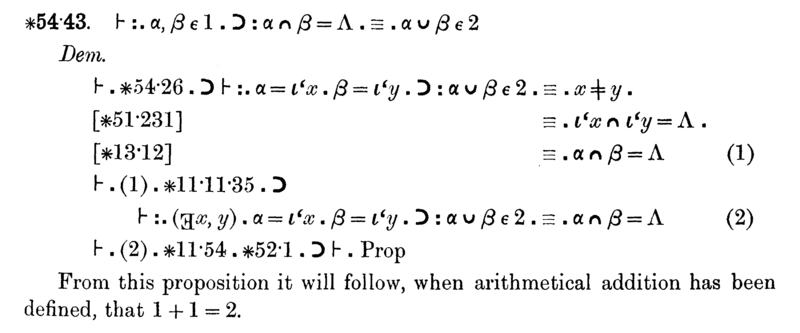The notation can be overwhelming, so let's focus just on the statement of the theorem, ignore everything else, even the helpful remark at the bottom: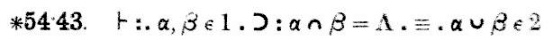This is the theorem that is being proved; what follows is the proof.

Now I should explain the notation, which has changed somewhat since 1910. First off, Principia Mathematica uses Peano's "dots" notation to disambiguate precedence, where we now use parentheses instead. The dot notation takes some getting used to, but has some distinct advantages over the parentheses. The idea is just that you indicate grouping by putting in dots, so that (1+2)×(3+4)×(5+6) is written as 1+2.×.3+4.×.5+6. The middle sub-formula is between a pair of dots. The (1+2) sub-formula is between a pair of dots also, but the dot on the left end is superfluous, and we omit it; similarly, the sub-formula (5+6) is delimited by a dot on the left and by the end of the formula on the right.

What if you need to nest parentheses? Then you use more dots. A double dot (:) is like a single dot, but stronger. For example, we write ((1+2)×3)+4 as 1+2 . × 3 : + 4, and the double dot isolates the entire 1+2 . × 3 expression into a single sub-formula to which the +4 applies.

Sometimes you need more levels of precedence, and then you use triple dots (.: and :.) and quadruple (::). This formula, as you see, has double and triple dots. Translating the dots into standard parenthesis notation, we have $$\ast54.43. \vdash ((\alpha, \beta \in 1 ) \supset (( \alpha\cap\beta = \Lambda) \equiv (\alpha\cup\beta \in 2)))$$. This is rather more cluttered-looking than the version with the dots, and in complicated formulas you can have trouble figuring out which parentheses match with which. With the dots, it's always easy. So I think it's a bit unfortunate that this convention has fallen out of use.

The !!\vdash!! symbol has not changed; it means that the formula to which it applies is asserted to be true. !!\supset!! is logical implication, and !!\equiv!! is logical equivalence. Λ is the empty set, which we write nowadays as ∅. ∩ ∪ and ∈ have their modern meanings: ∩ and ∪ are the set intersection and the union operators, and xy means that x is an element of set y.

The remaining points are semantic. α and β are sets. 1 denotes the set of all sets that have exactly one element. That is, it's the set { c : there exists a such that c = { a } }. Theorems about 1 include, for example:

• that Λ∉1 (∗52.21),

• that if α∈1 then there is some x such that α = {x} (∗52.1), and

• that {x}∈1 (∗52.22).

2 is similarly the set of all sets that have exactly two elements. An important theorem about 2 is ∗54.3, which says $$\ast54.3. \vdash 2 = \hat\alpha\{ (\exists x) \> .\> x\in\alpha \> . \> \alpha - \iota`x\in 1 \}.$$ In Principia Mathematica notation, {x}, the set that contains x and nothing else, is written ι‘x, so this theorem says that 2 is identical with the set of all α such that α has some element x , which, when removed from α, leaves a 1-element set.

So here is theorem ∗54.43 again:It asserts that if sets α and β each have exactly one element, then they are disjoint (that is, have no elements in common) if and only if their union has exactly two elements.

The proof, which appears in the scan above following the word "Dem." (short for "demonstration") goes like this: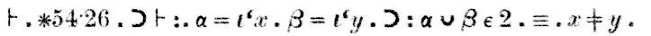"Theorem ∗54.26 implies that if α = {x} and β = {y}, then α∪β has 2 elements if and only if x is different from y."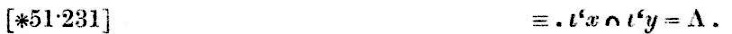"By theorem ∗51.231, this last bit (x is different from y) is true if and only if {x} and {y} are disjoint."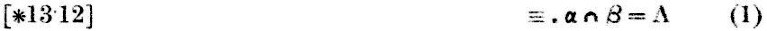"By ∗13.12, this last bit ({x} and {y} are disjoint) is true if and only if α and β themselves are disjoint." The partial conclusion at this point, which is labeled (1), is that if α = {x} and β = {y}, then α∪β ∈ 2 if and only if α∩β = Λ.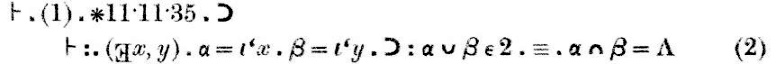The proof continues: "Conclusion (1), with theorems ∗11.11 and ∗11.35, implies that if there exists x and y so that α is {x} and β is {y}, then α∪β ∈ 2 if and only if α and β are disjoint." This conclusion is labeled (2).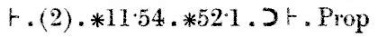Finally, conclusion (2), together with theorems ∗11.54 and ∗52.1, implies the theorem we were trying to prove.

Maybe the thing to notice here is how very small the steps are. ∗54.26, on which this theorem heavily depends, is almost the same; it asserts that {x}∪{y} ∈ 2 if and only if xy. ∗54.26, in turn, depends on ∗54.101, which says that α has 2 elements if and only if there exist x and y, not the same, such that α = {x} ∪ {y}. ∗54.101 is just a tiny bit different from the definition of 2. Theorem ∗51.231 says that {x} and {y} are disjoint if and only if x and y are different. ∗52.1 is a basic property of 1; we saw it before.

The other theorems cited in the demonstration are very tiny technical matters. ∗11.54 says that you can take an assertion that two things exist and separate it into two assertions, each one asserting that one of the things exists. ∗11.11 is even slimmer: it says that if φ(x, y) is always true, then you can attach a universal quantifier, and assert that φ(x, y) is true for all x and y. ∗13.12 concerns the substitution of equals for equals: if x and y are the same, then x possesses a property ψ if and only if y does too.

I haven't seen the later parts of Principia Mathematica, because my copy stops after section ∗56, and the arithmetic stuff is much later. But this theorem clearly has the sense of 1+1=2 in it, and the later theorem (∗110.643) that actually asserts 1+1=2 depends strongly on this one.

Although I am not completely sure what is going to happen later on (I've wasted far too much time on this already to put in more time to get the full version from the library) I can make an educated guess. Principia Mathematica is going to define the number 17 as being the set of all 17-element sets, and similarly for every other number; the use of the symbol 2 to represent the set of all 2-element sets prefigures this. These sets-of-all-sets-of-a-certain-size will then be identified as the "cardinal numbers".

The Principia Mathematica will define the sum of cardinal numbers p and q something like this: take a representative set a from p; a has p elements. Take a representative set b from q; b has q elements. Let c = ab. If c is a member of some cardinal number r, and if a and b are disjoint, then the sum of p and q is r.

With this definition, you can prove the usual desirable properties of addition, such as x + 0 = x, x + y = y + x, and 1 + 1 = 2.

In particular, 1+1=2 follows directly from theorem ∗54.43; it's just what we want, because to calculate 1+1, we must find two disjoint representatives of 1, and take their union; ∗54.43 asserts that the union must be an element of 2, regardless of which representatives we choose, so that 1+1=2.

Post scriptum: Peter Norvig says that the circumflex in the Principia Mathematica notation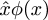is the ultimate source of the use of the word lambda to denote an anonymous function in the Lisp and Python programming languages. I am sure you know that these languages get "lambda" from the use of the Greek letter λ by Alonzo Church to represent function abstraction in his "λ-calculus": In Lisp, (lambda (u) B) is a function that takes an argument u and returns the value of B; in the λ-calculus, λu.B is a function that takes an argument u and returns the value of B. (Recall also that Church was the doctoral advisor of John McCarthy, the inventor of Lisp.) Norvig says that Church was originally planning to write the function λu.B as û.B, but his printer could not do circumflex accents. So he considered moving the circumflex to the left and using a capital lambda instead: Λu.B. The capital Λ looked too much like logical and ∧, which was confusing, so he used lowercase lambda λ instead.

Post post scriptum: Everyone always says "Russell and Whitehead". Google results for "Russell and Whitehead" outnumber those for "Whitehead and Russell" by two to one, for example. Why? The cover and the title page say "Alfred North Whitehead and Bertrand Russell, F.R.S.". How and when did Whitehead lose out on top billing?

[ Addendum 20060913: I figured out how and when Whitehead lost out on top billing: 10 December 1950. ]

[ Addendum 20171107: W. Ethan Duckworth sent me a translation of this proposition into modern notation, which I discussed in a new article. ]

[ Addendum 20211028: The duplication of “calculus of classes” as “calculus of relations” was fixed the following year by Norbert Wiener. ]

[ Addendum 20230329: I discussed Wiener's invention of the ordered pair in 2021:   ]

[ Addendum 20230328: I guessed “Principia Mathematica will define the sum of cardinal numbers p and q something like this…”. This is indeed more or less how it goes. Cardinal addition is introduced in ∗110. The 1+1=2 theorem looks like this: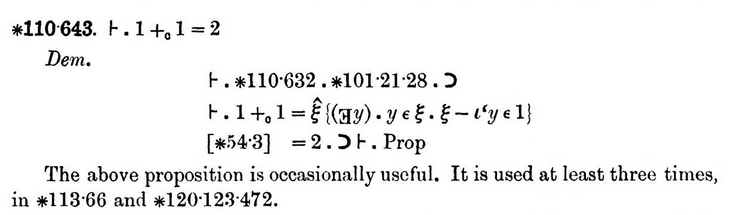The referenced ∗54.3, recall, is the definition of the number 2. The theorem x + y = y + x is ∗110.51, and x + 0 = x is ∗110.61. ]